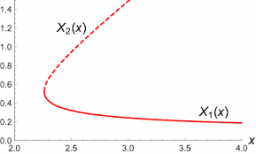# Combinations 70714

If we increase the number of elements by 1, the number of combinations of the third class without repetitions increases by 10. How many elements do we have?

n =  5

### Step-by-step explanation:Did you find an error or inaccuracy? Feel free to write us. Thank you!

Tips for related online calculators
Are you looking for help with calculating roots of a quadratic equation?
Need help calculating sum, simplifying, or multiplying fractions? Try our fraction calculator.
Would you like to compute the count of combinations?
Do you have a linear equation or system of equations and looking for its solution? Or do you have a quadratic equation?